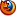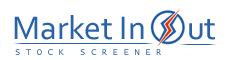Please enable JavaScript to view this page content properly Log In | Sign Up
 Pre-Tax Profit Margin Leverage Ratio Current Ratio Price / Book Ratio Price / Tangible Book Ratio Price / Cash Flow Ratio Price / Free Cash Flow Ratio Debt / Equity Ratio Price / Sales Ratio Income per Employee
 Price / Free Cash Flow Ratio Action Price / Free Cash Flow Ratio: More than 200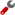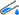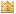Price / Free Cash Flow Ratio: 100 to 200Price / Free Cash Flow Ratio: 80 to 100Price / Free Cash Flow Ratio: 50 to 80Price / Free Cash Flow Ratio: 30 to 50Price / Free Cash Flow Ratio: 20 to 30Price / Free Cash Flow Ratio: 10 to 20Price / Free Cash Flow Ratio: 0 to 10Best viewed in